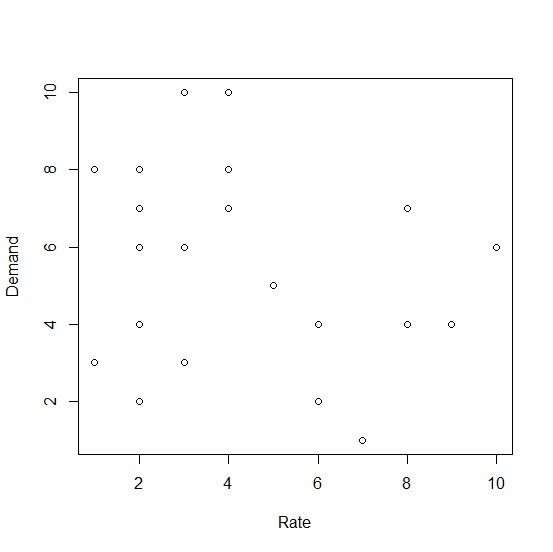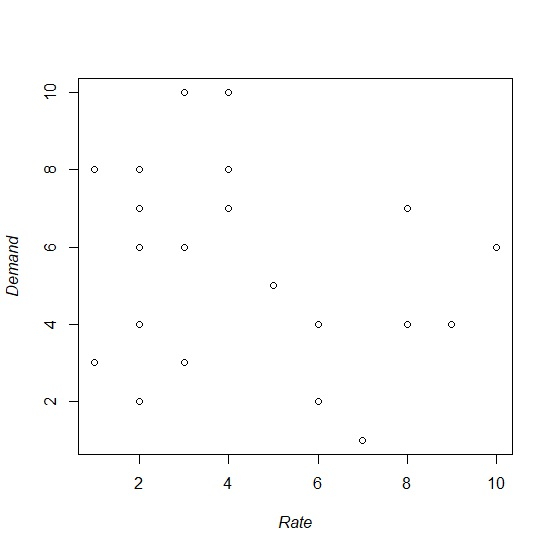# How to display base R plot axes titles in italics?

To display base R plot axes titles in italics, we can follow the below steps −

• First of all, create two vectors and plot them using plot function.

• Then, use expression function for ylab and xlab to change the axes titles into italics font.

## Create the vectors and plot them

Let’s create two vectors Rate and Demand and plot them using plot function −

Live Demo

Rate<-sample(1:10,25,replace=TRUE)
Demand<-sample(1:10,25,replace=TRUE)
plot(Rate,Demand)

On executing, the above script generates the below output(this output will vary on your system due to randomization) −

## Output## Convert axes titles to italics

Using expression function with italic function to convert the axes titles into italic font −

Live Demo

Rate<-sample(1:10,25,replace=TRUE)
Demand<-sample(1:10,25,replace=TRUE)
plot(Rate,Demand,ylab=expression(italic("Demand")),xlab=expression(italic("Rate")))

## Output